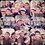# Value of Trigonometry of Special Angles

Let us start from the value of sin.

$$\sin{0} = \frac{1}{2}\sqrt{0}$$

$\sin{30} = \frac{1}{2}\sqrt{1}$

$\sin{45} = \frac{1}{2}\sqrt{2}$

$\sin{60} = \frac{1}{2}\sqrt{3}$

$\sin{90} = \frac{1}{2}\sqrt{4}$

$\sin{120} = \frac{1}{2}\sqrt{3}$

$\sin{135} = \frac{1}{2}\sqrt{2}$

$\sin{150} = \frac{1}{2}\sqrt{1}$

$\sin{180} = \frac{1}{2}\sqrt{0}$

$\sin{210} = -\frac{1}{2}\sqrt{1}$

$\sin{225} = -\frac{1}{2}\sqrt{2}$

$\sin{240} =- \frac{1}{2}\sqrt{3}$

$\sin{270} = -\frac{1}{2}\sqrt{4}$

$\sin{300} = -\frac{1}{2}\sqrt{3}$

$\sin{315} = -\frac{1}{2}\sqrt{2}$

$\sin{330} = -\frac{1}{2}\sqrt{1}$

$\sin{360} = -\frac{1}{2}\sqrt{0}$

Let us continue with cos.

$\cos{0} = \frac{1}{2}\sqrt{4}$

$\cos{30} = \frac{1}{2}\sqrt{3}$

$\cos{45} = \frac{1}{2}\sqrt{2}$

$\cos{60} = \frac{1}{2}\sqrt{1}$

$\cos{90} = \frac{1}{2}\sqrt{0}$

$\cos{120} = -\frac{1}{2}\sqrt{1}$

$\cos{135} = -\frac{1}{2}\sqrt{2}$

$\cos{150} = -\frac{1}{2}\sqrt{3}$

$\cos{180} = -\frac{1}{2}\sqrt{4}$

$\cos{210} = -\frac{1}{2}\sqrt{3}$

$\cos{225} = -\frac{1}{2}\sqrt{2}$

$\cos{240} = -\frac{1}{2}\sqrt{1}$

$\cos{270} = \frac{1}{2}\sqrt{0}$

$\cos{300} = \frac{1}{2}\sqrt{1}$

$\cos{315} = \frac{1}{2}\sqrt{2}$

$\cos{330} = \frac{1}{2}\sqrt{3}$

$\cos{360} = \frac{1}{2}\sqrt{4}$

For the value of tan, cot, sec, and csc, just remember that

$\tan \theta = \frac{\sin \theta}{\cos \theta}$

$\cot \theta = \frac{1}{\tan \theta}$

$\sec \theta = \frac{1}{\cos \theta}$

$\csc \theta = \frac{1}{\sin \theta}$

$\sin{15} = \frac{\sqrt{6}-\sqrt{2}}{4}$

$\sin{75} = \frac{\sqrt{6}+\sqrt{2}}{4}$

$\sin{105} = \frac{\sqrt{6}+\sqrt{2}}{4}$

$\sin{165} = \frac{\sqrt{6-}\sqrt{2}}{4}$

$\sin{195} = \frac{-\sqrt{6}+\sqrt{2}}{4}$

$\sin{255} = -\frac{\sqrt{6}+\sqrt{2}}{4}$

$\sin{285} = -\frac{\sqrt{6}+\sqrt{2}}{4}$

$\sin{345} = \frac{-\sqrt{6}+\sqrt{2}}{4}$

$\cos{15} = \frac{\sqrt{6}+\sqrt{2}}{4}$

$\cos{75} = \frac{\sqrt{6}-\sqrt{2}}{4}$

$\cos{105} = \frac{-\sqrt{6}+\sqrt{2}}{4}$

$\cos{165} = -\frac{\sqrt{6}+\sqrt{2}}{4}$

$\cos{195} = -\frac{\sqrt{6}+\sqrt{2}}{4}$

$\cos{255} = \frac{-\sqrt{6}+\sqrt{2}}{4}$

$\cos{285} = \frac{\sqrt{6}-\sqrt{2}}{4}$

$\cos{345} = \frac{\sqrt{6}+\sqrt{2}}{4}$Note by Jonathan Christianto
6 years, 6 months ago

This discussion board is a place to discuss our Daily Challenges and the math and science related to those challenges. Explanations are more than just a solution — they should explain the steps and thinking strategies that you used to obtain the solution. Comments should further the discussion of math and science.

When posting on Brilliant:

• Use the emojis to react to an explanation, whether you're congratulating a job well done , or just really confused .
• Ask specific questions about the challenge or the steps in somebody's explanation. Well-posed questions can add a lot to the discussion, but posting "I don't understand!" doesn't help anyone.
• Try to contribute something new to the discussion, whether it is an extension, generalization or other idea related to the challenge.

MarkdownAppears as
*italics* or _italics_ italics
**bold** or __bold__ bold
- bulleted- list
• bulleted
• list
1. numbered2. list
1. numbered
2. list
Note: you must add a full line of space before and after lists for them to show up correctly
paragraph 1paragraph 2

paragraph 1

paragraph 2

[example link](https://brilliant.org)example link
> This is a quote
This is a quote
    # I indented these lines
# 4 spaces, and now they show
# up as a code block.

print "hello world"
# I indented these lines
# 4 spaces, and now they show
# up as a code block.

print "hello world"
MathAppears as
Remember to wrap math in $$ ... $$ or $ ... $ to ensure proper formatting.
2 \times 3 $2 \times 3$
2^{34} $2^{34}$
a_{i-1} $a_{i-1}$
\frac{2}{3} $\frac{2}{3}$
\sqrt{2} $\sqrt{2}$
\sum_{i=1}^3 $\sum_{i=1}^3$
\sin \theta $\sin \theta$
\boxed{123} $\boxed{123}$

Sort by:

Very nice! I'd never seen them laid out that way before.

- 6 years, 6 months ago

Yes, sir. I got it from my teacher

- 6 years, 6 months ago